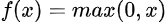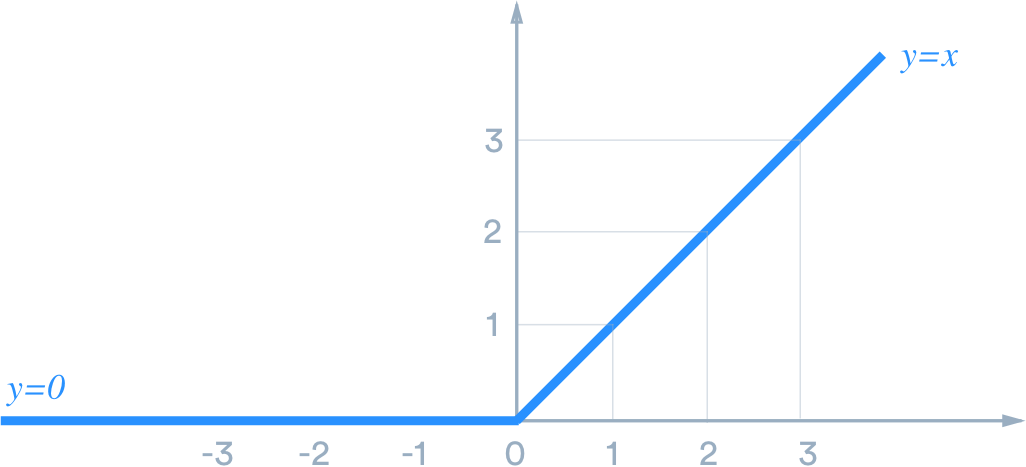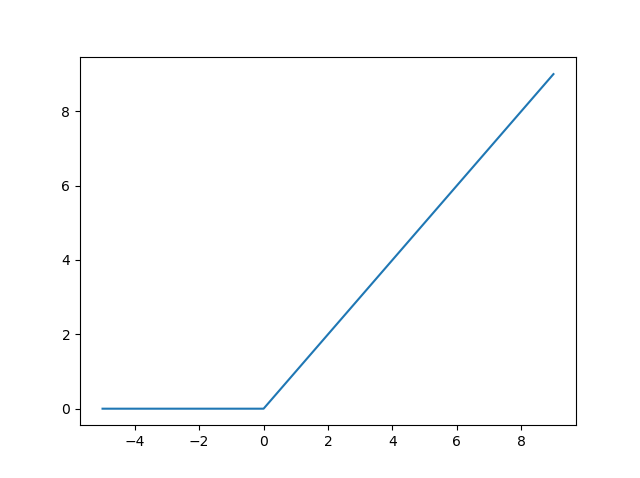×

Search anything:

# ReLU (Rectified Linear Unit) Activation Function

#### Machine Learning (ML)We will take a look at the most widely used activation function called ReLU (Rectified Linear Unit) and understand why it is preferred as the default choice for Neural Networks. This article tries to cover most of the important points about this function.

1. Brief Overview of Neural Networks
2. What is an activation function?
3. What is ReLU?
4. Implementing ReLU function in Python
5. Why is ReLU non-linear?
6. Derivative Of ReLU

Pre-requisites:

## Brief Overview of Neural Networks

Artificial Neural Networks are very much analogous to a human brain, they consist of various layers that perform a particular task. Each layer consists of various number of neurons which are similar to the biological neurons within the human body, they get activated under certain circumstances resulting in a related action performed by the body in response to stimuli. These neurons are inter-connected to various layers which are powered by activation functions.

Information is passed from one layer to the other through the process of forward propagation i.e from the input layer to the output layer. The loss function is calculated once the output variable is obtained. The back-propagation is done to update the weights and reduce the loss function with the help of an optimizer - the most common optimizer algorithm is gradient descent. Multiple epochs are run until the loss converges to the global minima.

## What is an activation function?

Activation function is a simple mathematical function that transforms the given input to the required output that has a certain range. From their name they activate the neuron when output reaches the set threshold value of the function. Basically they are responsible for switching the neuron ON/OFF. The neuron recieves the sum of the product of inputs and randomly initialized weights along with a static bias for each layer. The activation function is applied on to this sum, and an output is generated. Activation functions introduce a non-linearity, so as to make the network learn complex patterns in the data such as in the case of images, text, videos or sounds. Without an activation function our model is going to behave like a linear regression model that has limited learning capacity.

## What is ReLU ?

The rectified linear activation function or ReLU is a non-linear function or piecewise linear function that will output the input directly if it is positive, otherwise, it will output zero.
It is the most commonly used activation function in neural networks, especially in Convolutional Neural Networks (CNNs) & Multilayer perceptrons.

It is simple yet it is more effective than it's predecessors like sigmoid or tanh.

Mathematically, it is expressed as:Graphically it is represented as,## Implementing ReLU function in Python

We can implement a simple ReLU function with Python code using an if-else statement as,

def ReLU(x):
if x>0:
return x
else:
return 0


or using the max() in-built function over the range from 0.0 to x:

def relu(x):
return max(0.0, x)


The positive value is returned as it is and for values less than (negative values) or equal to zero, 0.0 is returned.

Now, we'll test out function by giving some input values and plot our result
using pyplot from matplotlib library. The input range of values is from -5 to 10. We apply our defined function on this set of input values.

from matplotlib import pyplot

def relu(x):
return max(0.0, x)

input = [x for x in range(-5, 10)]
# apply relu on each input
output = [relu(x) for x in input]
# plot our result
pyplot.plot(series_in, series_out)
pyplot.show()We see from the plot that all the negative values have been set to zero, and the positive values are returned as it is. Note that we've given a set of consecutively increasing numbers as input, so we've a linear output with an increasing slope.

## Why is ReLU non-linear?

At the first glance after plotting ReLU it seems to be a linear function. But in fact, it is a non-linear function and it is required so as to pick up & learn complex relationships from the training data.

It acts as a linear function for positive values and as a non-linear activation function for negative values.

When we're using an optimizer such as SGD (Stochastic Gradient Descent) during backpropagation, it acts like a linear function for positive values and thus it becomes a lot easier when computing the gradient. This near linearity allows to preserve properties and makes linear models easy to be optimized with gradient based algorithms.

Also ReLU adds more sensitivity to weighted sum and thus this avoids neurons from getting saturated (i.e when there is little or no variation in the output).

## Derivative Of ReLU:

The derivative of an activation function is required when updating the weights during the backpropagation of the error. The slope of ReLU is 1 for positive values and 0 for negative values. It becomes non-differentiable when the input x is zero, but it can be safely assumed to be zero and causes no problem in practice.

ReLU is used in the hidden layers instead of Sigmoid or tanh as using sigmoid or tanh in the hidden layers leads to the infamous problem of "Vanishing Gradient". The "Vanishing Gradient" prevents the earlier layers from learning important information when the network is backpropagating. The sigmoid which is a logistic function is more preferrable to be used in regression or binary classification related problems and that too only in the output layer, as the output of a sigmoid function ranges from 0 to 1. Also Sigmoid and tanh saturate and have lesser sensitivity.

Some of the advantages of ReLU are:

• Simpler Computation: Derivative remains constant i.e 1 for a positive input and thus reduces the time taken for the model to learn and in minimizing the errors.
• Representational Sparsity: It is capable of outputting a true zero value.
• Linearity: Linear activation functions are easier to optimize and allow for a smooth flow. So, it is best suited for supervised tasks on large sets of labelled data.

• Exploding Gradient: This occurs when the gradient gets accumulated, this causes a large differences in the subsequent weight updates. This as a result causes instability when converging to the global minima and causes instability in the learning too.
• Dying ReLU: The problem of "dead neurons" occurs when the neuron gets stuck in the negative side and constantly outputs zero. Because gradient of 0 is also 0, it's unlikely for the neuron to ever recover. This happens when the learning rate is too high or negative bias is quite large.

With this article at OpenGenus, you must have the complete idea of ReLU (Rectified Linear Unit) Activation Function.#### Vivek Praharsha

ML & DL Enthusiast

ReLU (Rectified Linear Unit) Activation Function